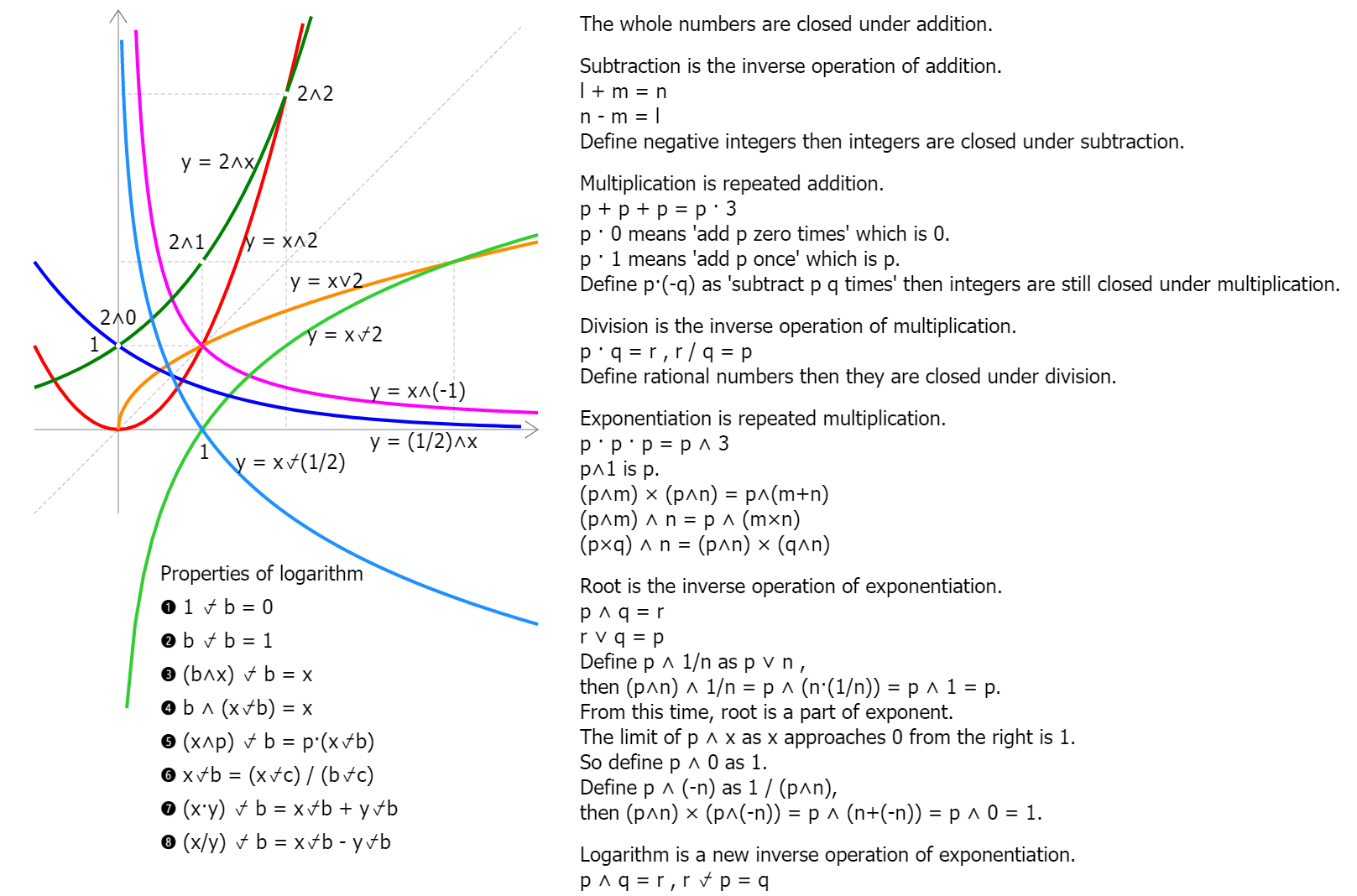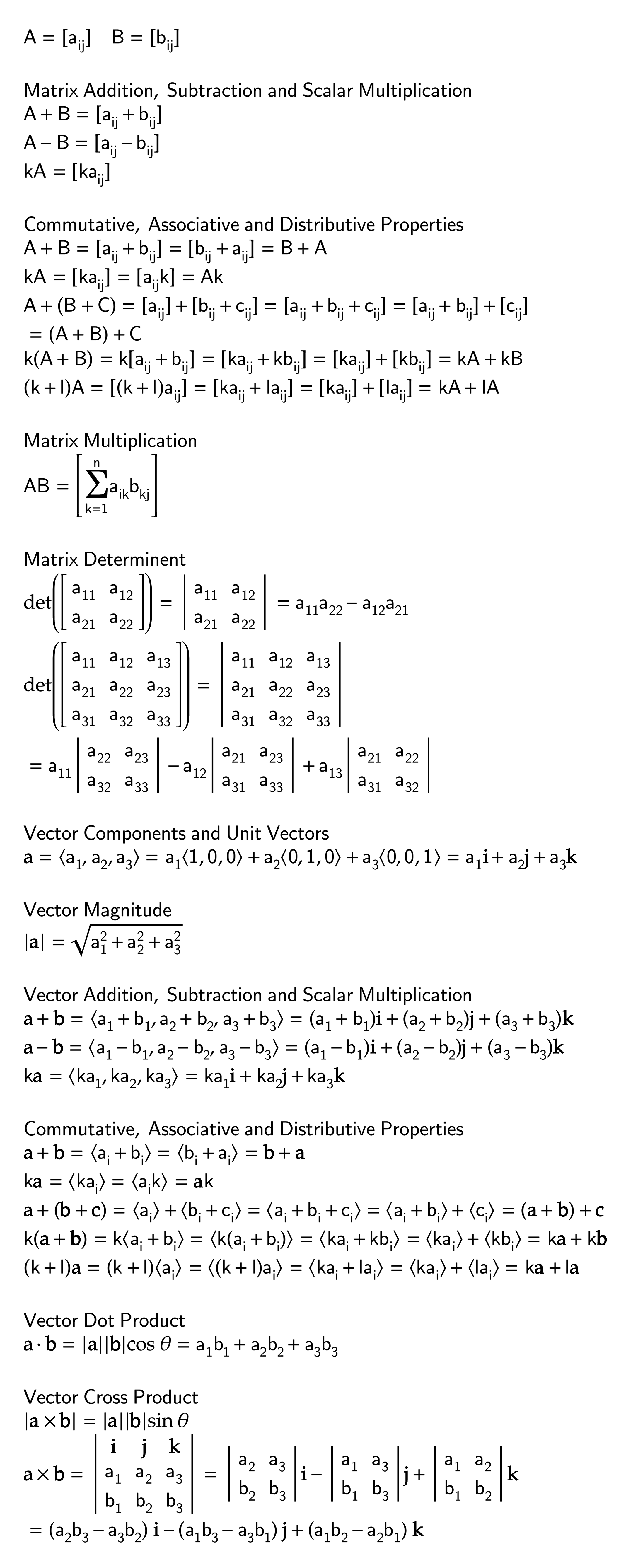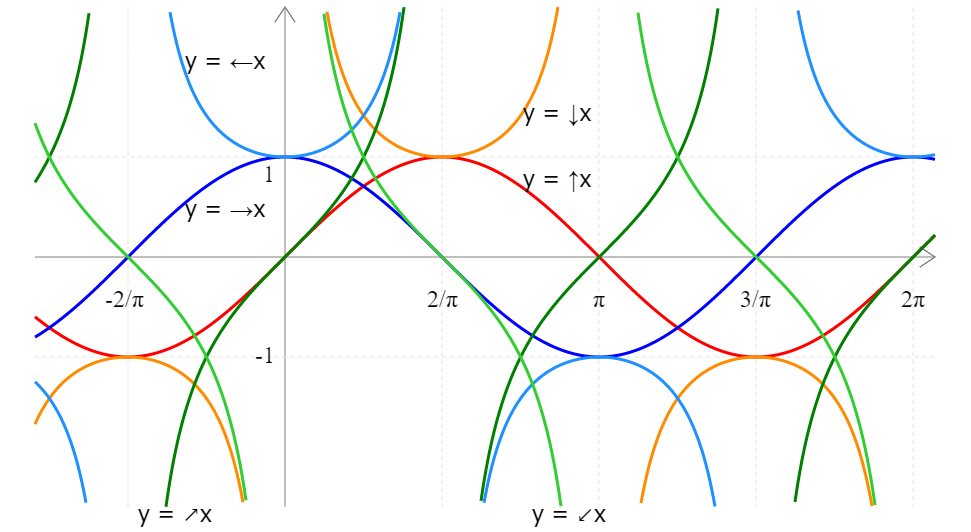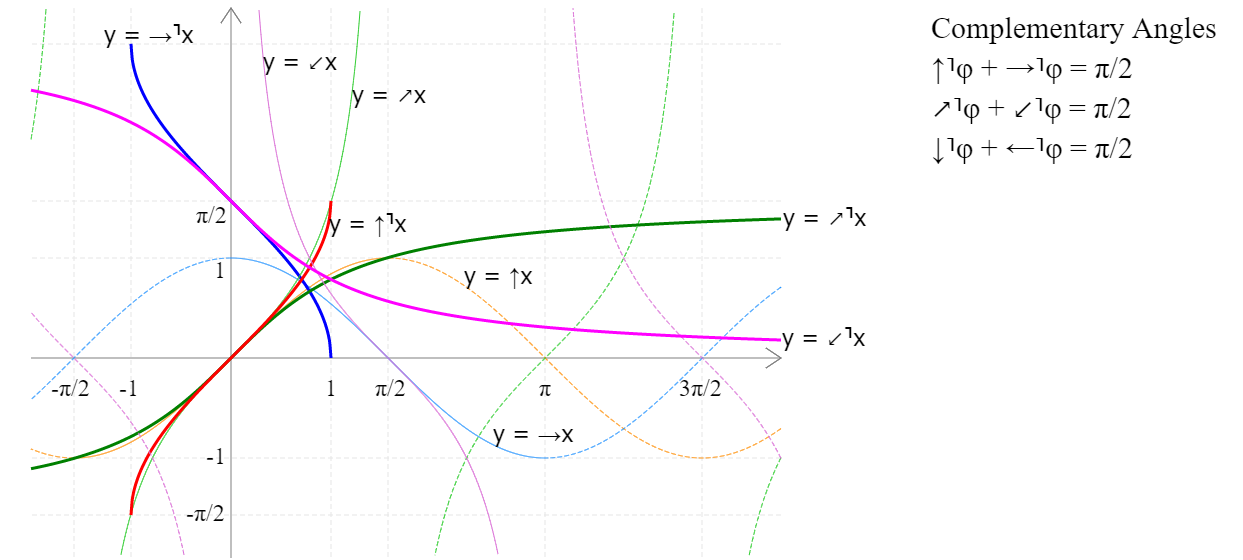EngliSea > math

arithmetic, geometry, algebra, exponentiation, logarithm, trigonometry, calculus, topologyMap of Mathematics
3676

00 History
In mathematics, the notion of number has been extended over the centuries to include 0, negative numbers, rational numbers such as 1/2 and −2/3, real numbers such as √2 and π, and complex numbers, which extend the real numbers with √−1.
4758#5148

10 Arithmatic
The study of numbers, especially the properties of the traditional operations on them—addition, subtraction, multiplication and division.
4758#481615 2D shapes
955315 3D shapes
9546

15 Geometry
A branch of mathematics concerned with questions of shape, size, relative position of figures, and the properties of space
4758#499920 Linear Equations
965120 Algebra properties
9552

20 Algebra
The study of mathematical symbols and the rules for manipulating these symbols
4758#498525 Expansion of Operators and Numbers
3694 COMMENT

25 Exponent, root and logarithm
Exponentiation is repeated multiplication. Root is the inverse operation of exponentiation. Logarithm is a new inverse operation of exponentiation.
4758#521630 2 Dimensional Number
9657

30 Complex Number
The set C of complex numbers is the set R2 of ordered pairs of real numbers. a+bi = [a,b]
4758#960535 Linear Algebra
3678 COMMENT

35 Linear Algebra
The branch of mathematics concerning linear equations, linear maps and their representations in vector spaces and through matrices.
4758#509350 unit circle
367550 reciprocal functions
3671 COMMENT50 inverse functions
6086 COMMENT50 Trigonometric laws and identities
3855

50 Trigonometry
A branch of mathematics that studies relationships between side lengths and angles of triangles
4758#5019

55 Analysis
Fundamentals of Mathematical Analysis
4758#970260 Calculus - derivatives and limits
373160 Calculus - integrals
3698

60 Calculus
Whether differential or integral both concepts involve the idea that we can do something infinately many times and get a finite answer that is useful.
4758#5028

70 Topology
The mathematical study of the properties that are preserved through deformations, twistings, and stretchings of objects
4758#5233

￤terms
•••
4758#5079-